# R S Aggarwal Solutions for Class 11 Maths Chapter 26 Three Dimensional Geometry Exercise 26B

R S Aggarwal Solutions for Class 11 Maths are given here for Chapter 26 exercise 26B. This exercise in RS Aggarwal Solutions is based on distance formula, which states that,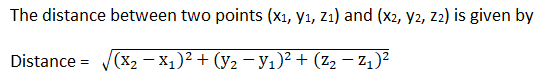In this exercise, students will learn to find the distance between two given points and solve various problems related to it. For example, distance formula helps to check if any three given points form an isosceles right-angled triangle or not.

Check the detailed R S Aggarwal solutions for class 11 chapter 26 exercise 26B below.

## Download PDF of R S Aggarwal Solutions for Class 11 Maths Chapter 26 Three Dimensional Geometry Exercise 26B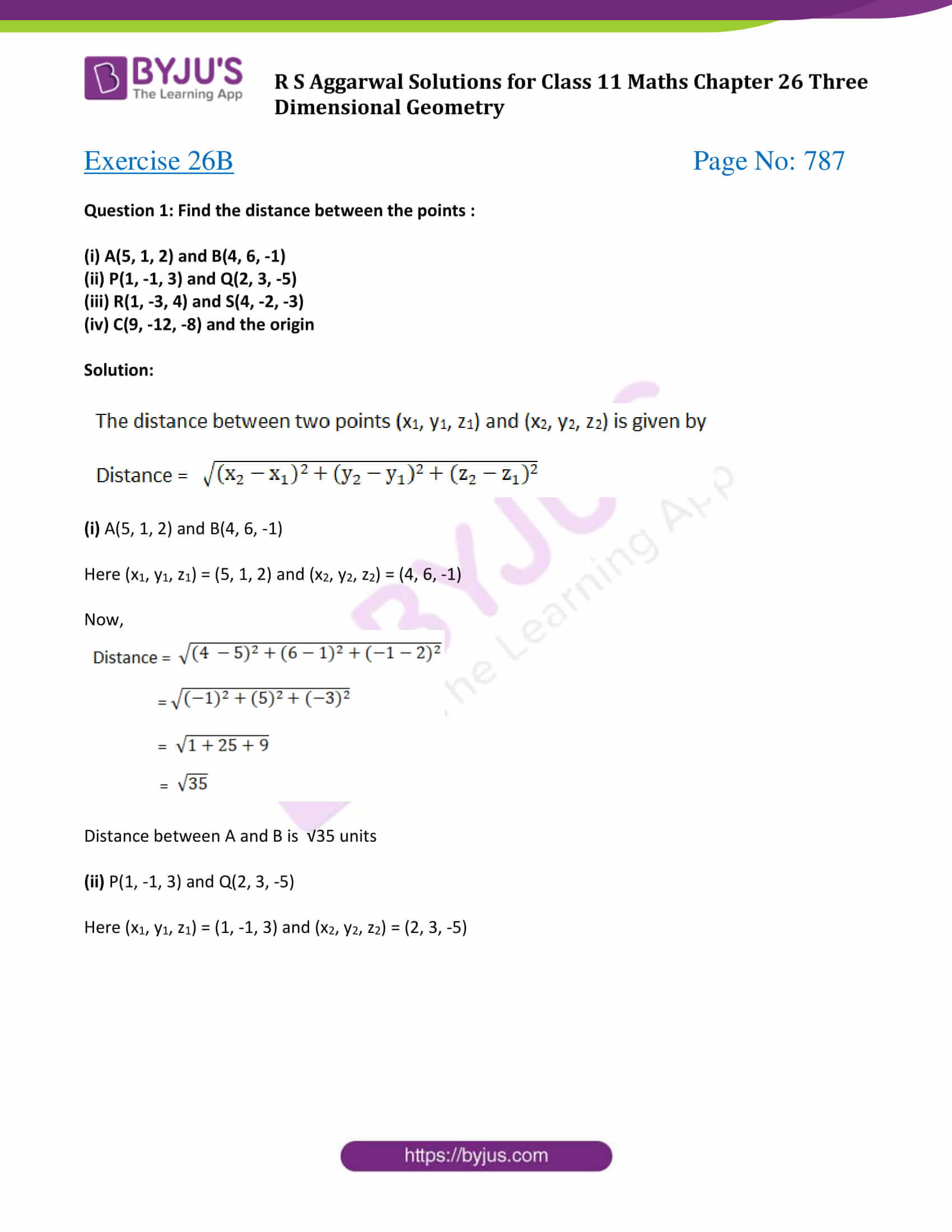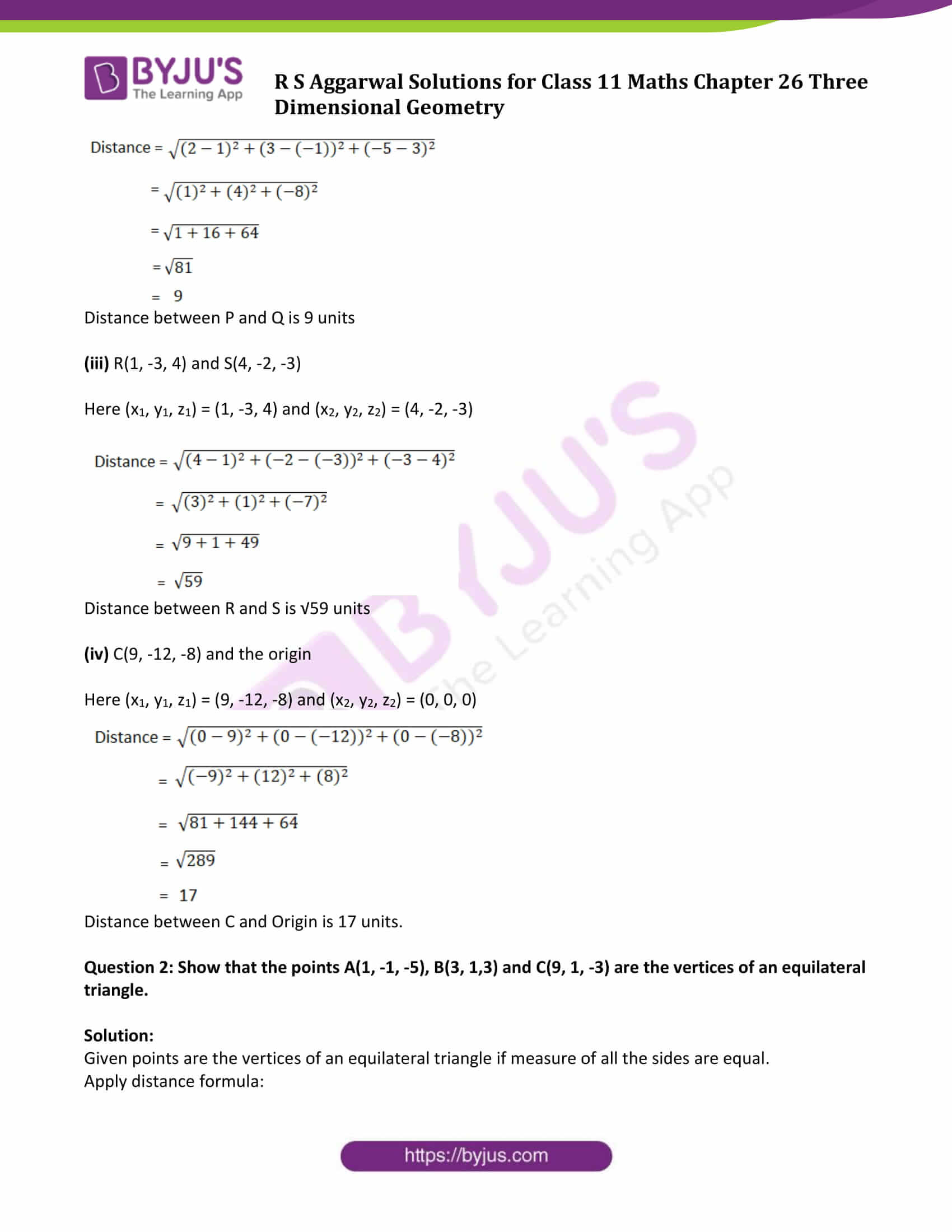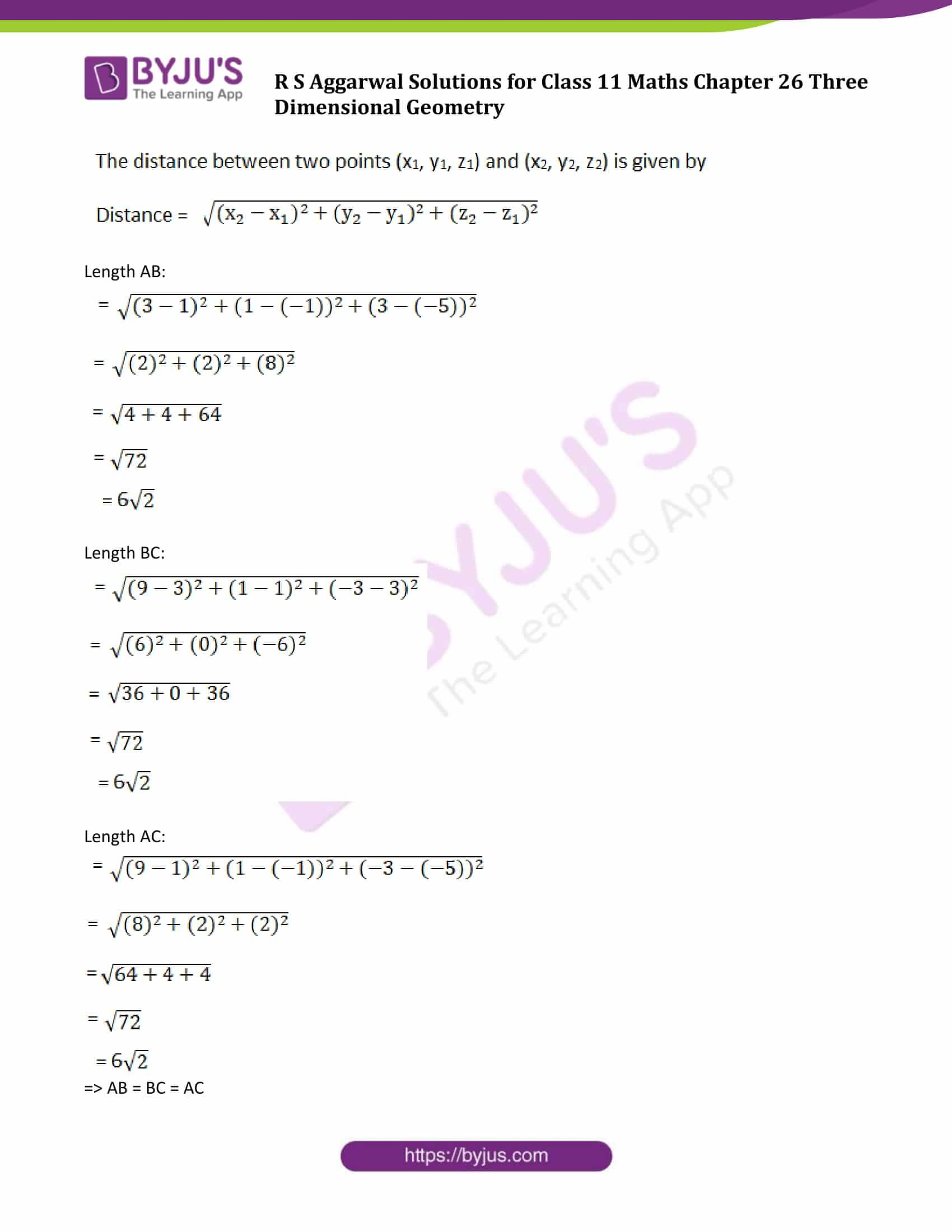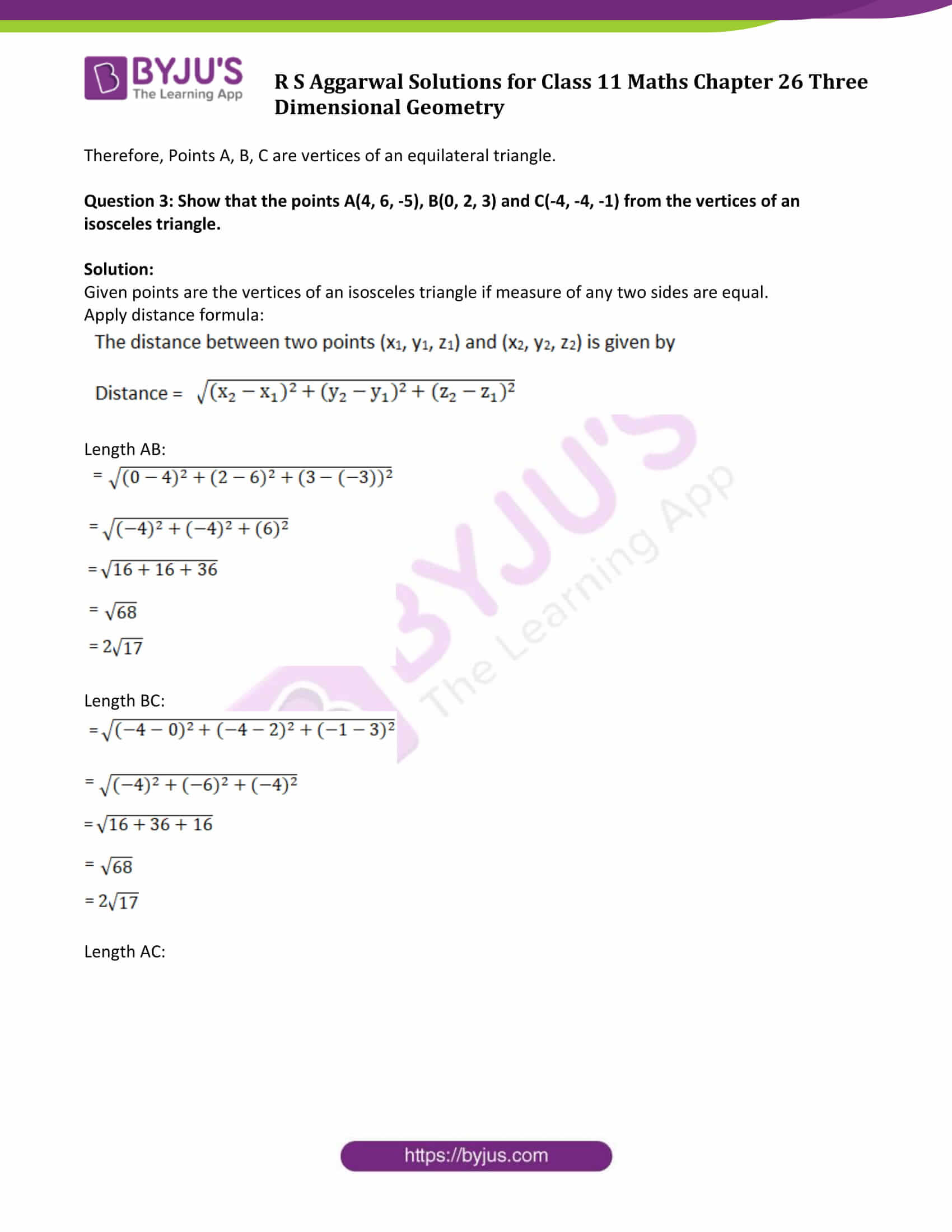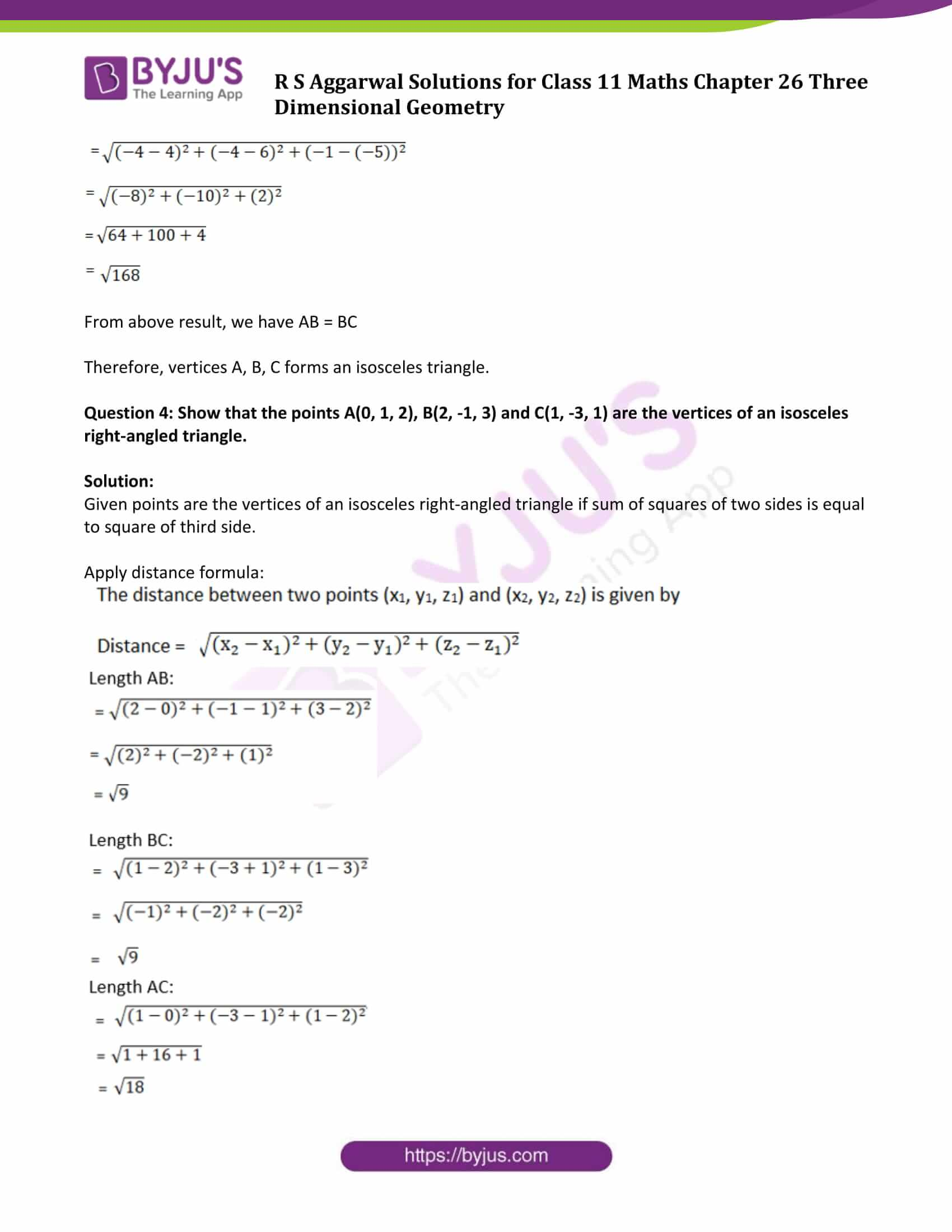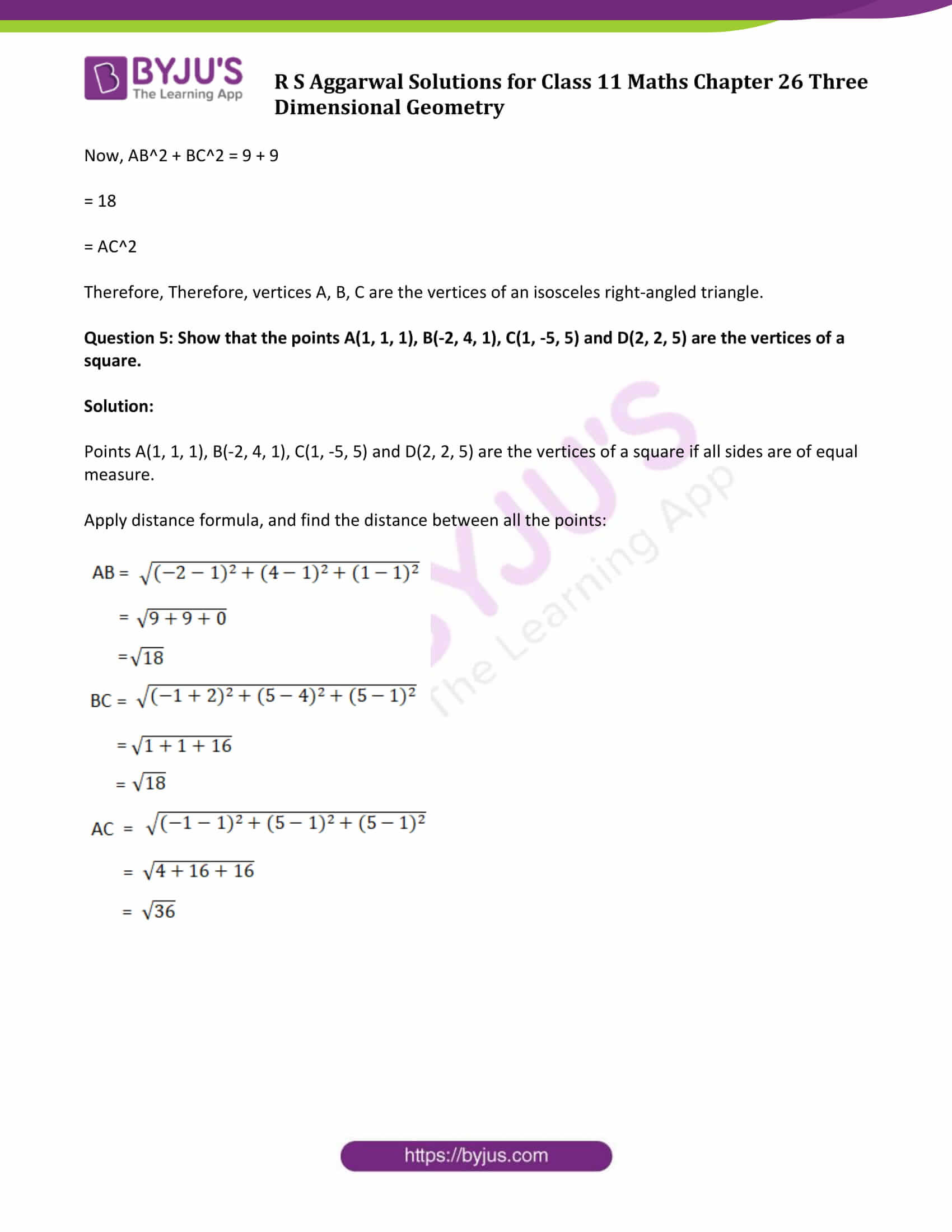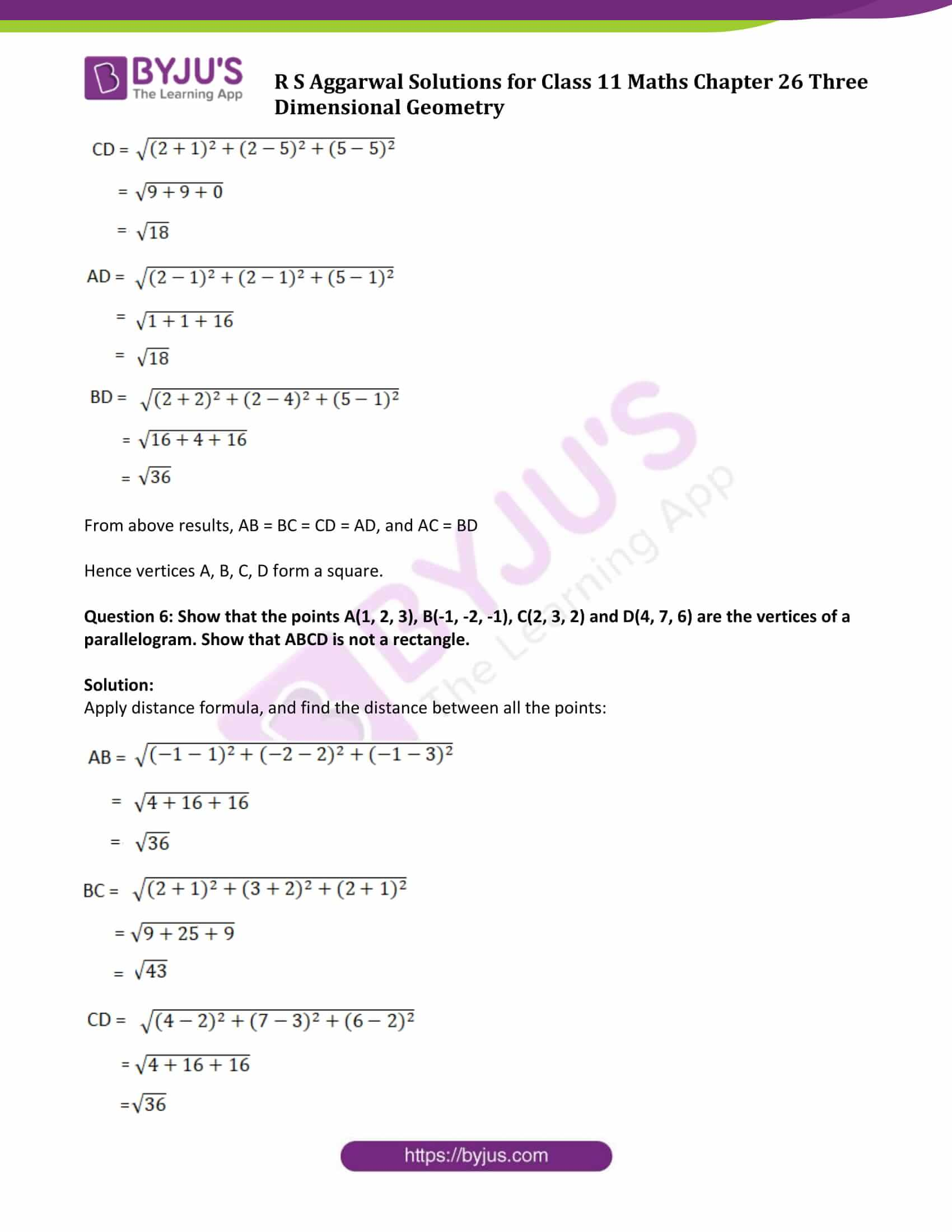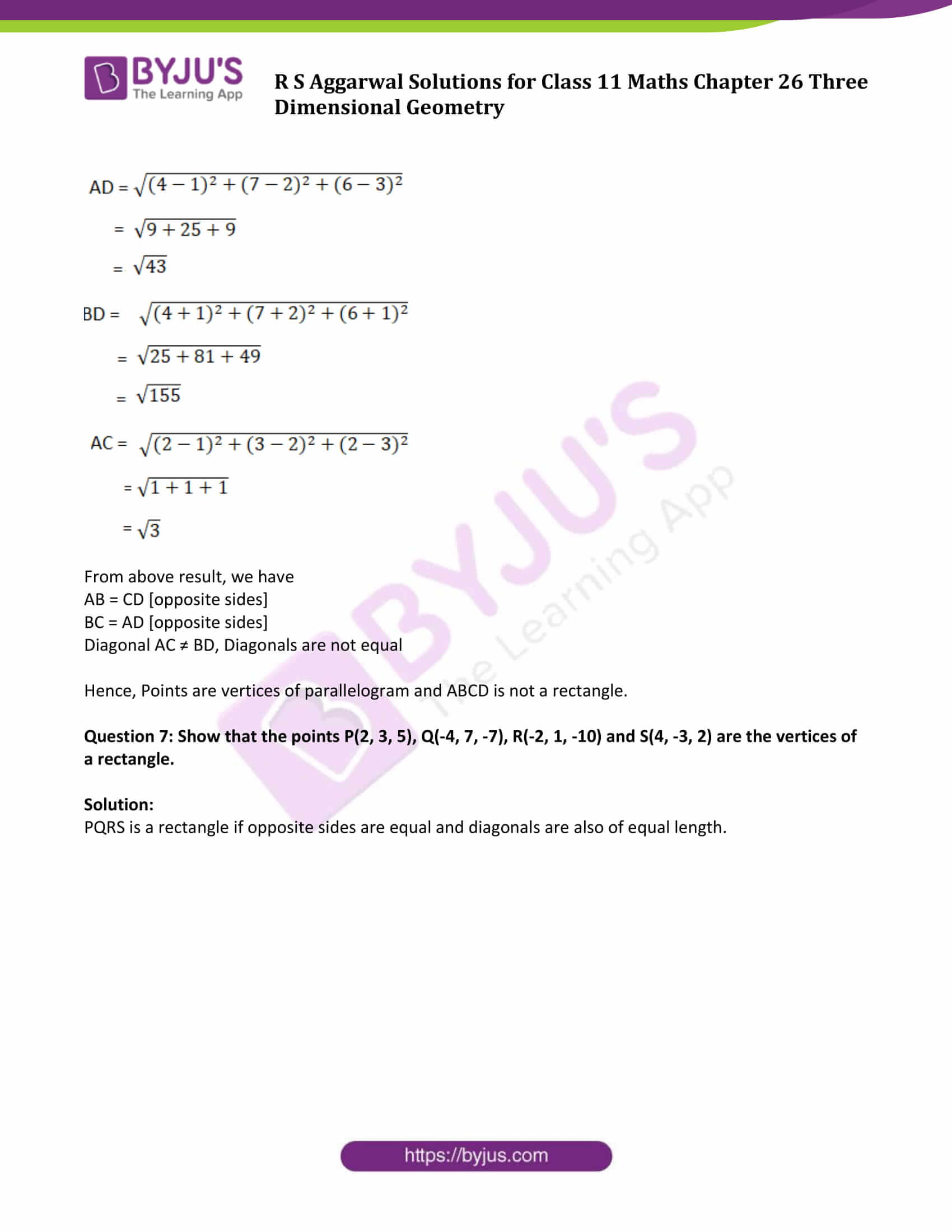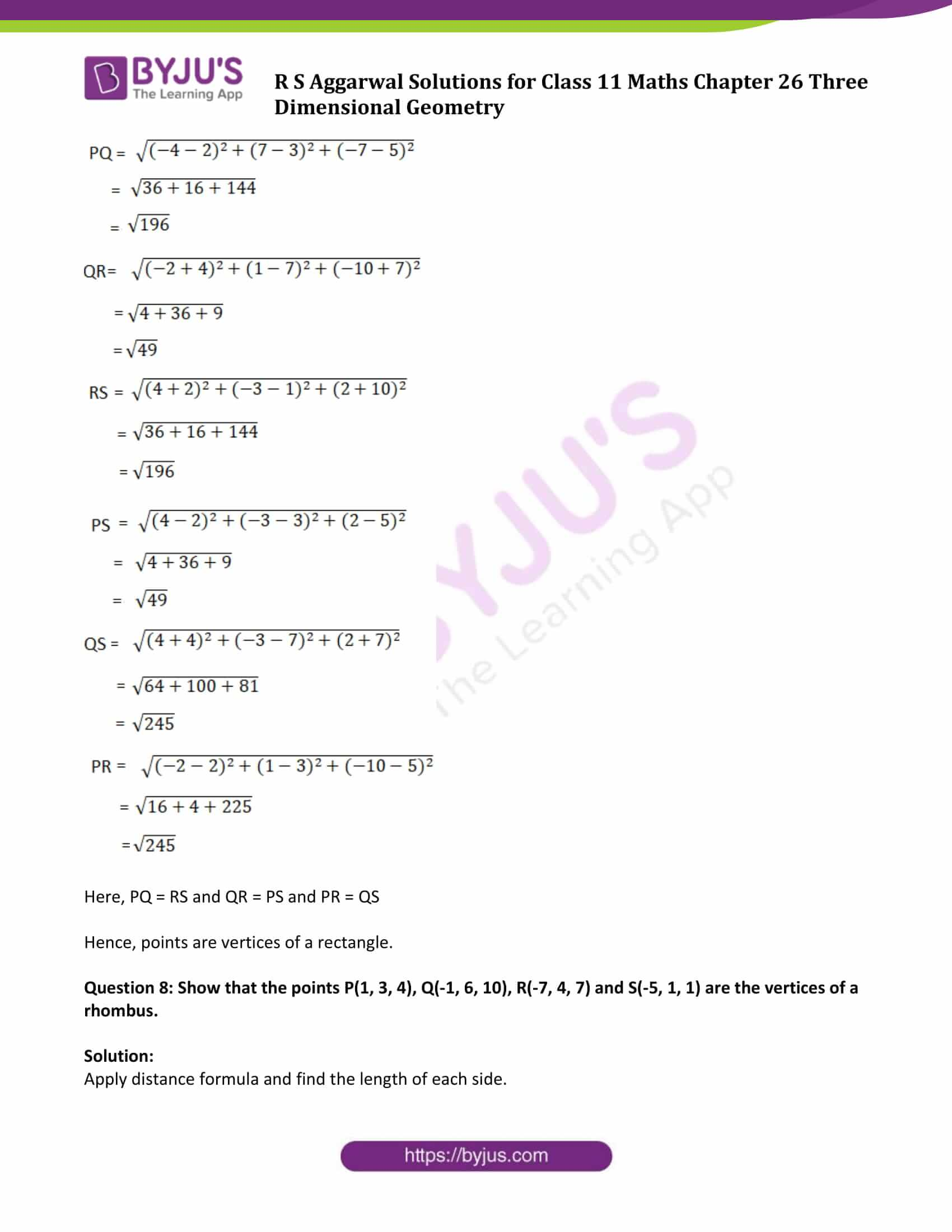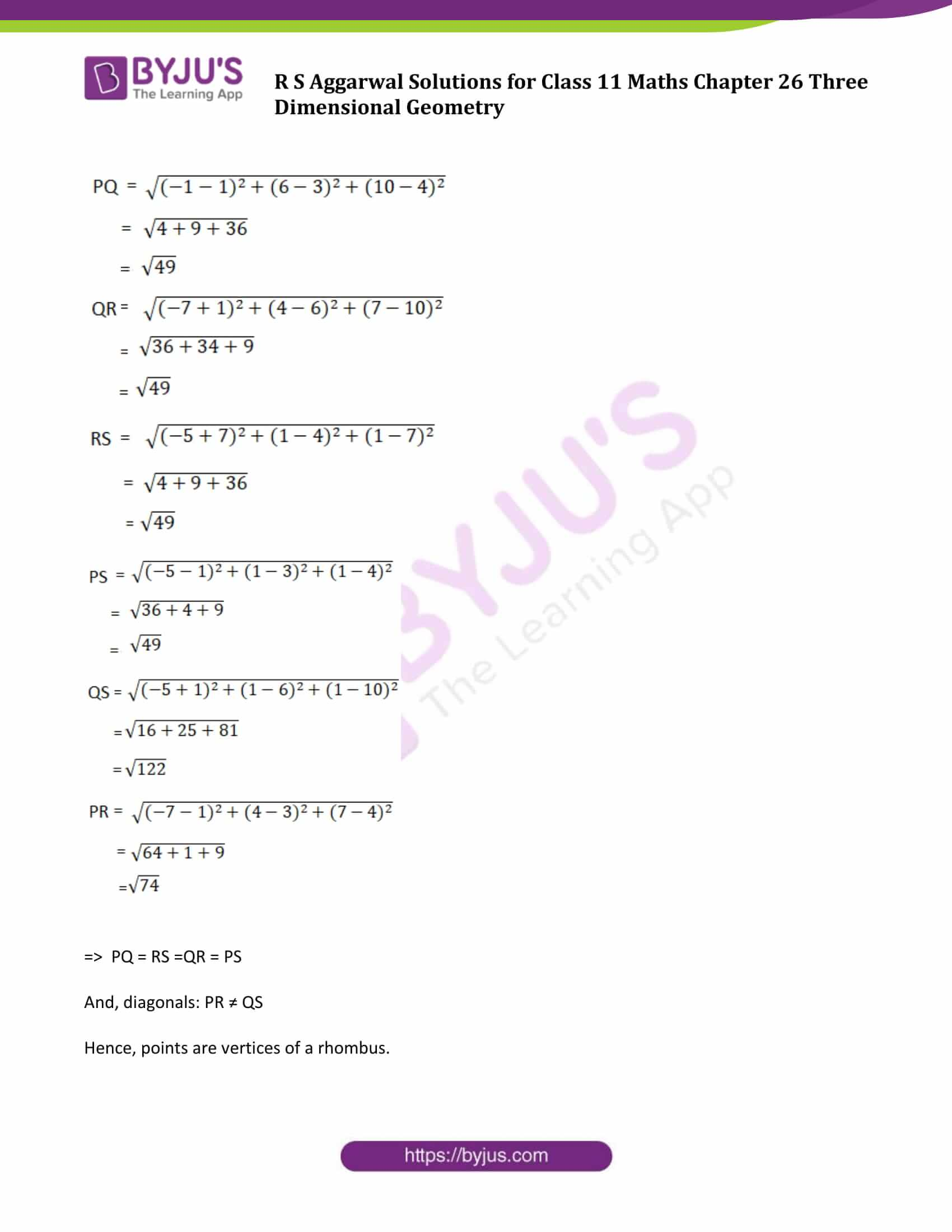### Access Answers of R S Aggarwal Solutions for Class 11 Maths Chapter 26 Three Dimensional Geometry Exercise 26B Page number 787

Exercise 26B Page No: 787

Question 1: Find the distance between the points :

(i) A(5, 1, 2) and B(4, 6, -1)

(ii) P(1, -1, 3) and Q(2, 3, -5)

(iii) R(1, -3, 4) and S(4, -2, -3)

(iv) C(9, -12, -8) and the origin

Solution:(i) A(5, 1, 2) and B(4, 6, -1)

Here (x1, y1, z1) = (5, 1, 2) and (x2, y2, z2) = (4, 6, -1)

Now,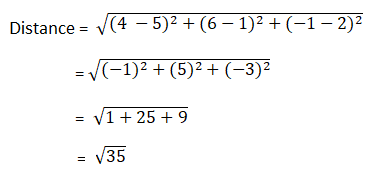Distance between A and B is √35 units

(ii) P(1, -1, 3) and Q(2, 3, -5)

Here (x1, y1, z1) = (1, -1, 3) and (x2, y2, z2) = (2, 3, -5)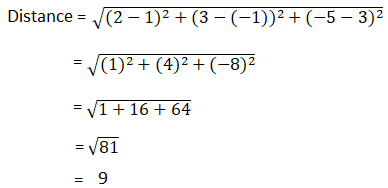Distance between P and Q is 9 units

(iii) R(1, -3, 4) and S(4, -2, -3)

Here (x1, y1, z1) = (1, -3, 4) and (x2, y2, z2) = (4, -2, -3)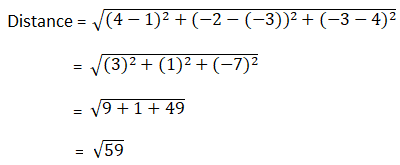Distance between R and S is √59 units

(iv) C(9, -12, -8) and the origin

Here (x1, y1, z1) = (9, -12, -8) and (x2, y2, z2) = (0, 0, 0)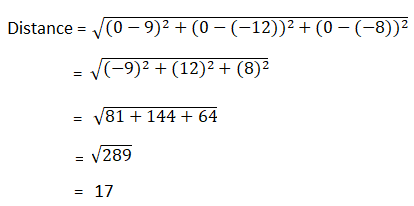Distance between C and Origin is 17 units.

Question 2: Show that the points A(1, -1, -5), B(3, 1,3) and C(9, 1, -3) are the vertices of an equilateral triangle.

Solution:

Given points are the vertices of an equilateral triangle if measure of all the sides are equal.

Apply distance formula:Length AB: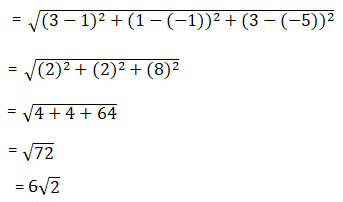Length BC: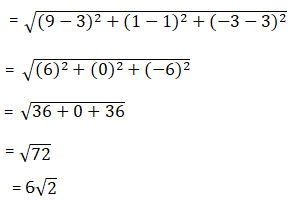Length AC: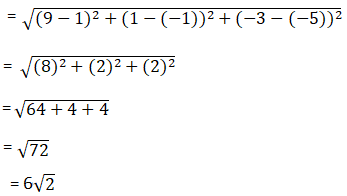=> AB = BC = AC

Therefore, Points A, B, C are vertices of an equilateral triangle.

Question 3: Show that the points A(4, 6, -5), B(0, 2, 3) and C(-4, -4, -1) from the vertices of an isosceles triangle.

Solution:

Given points are the vertices of an isosceles triangle if measure of any two sides are equal.

Apply distance formula:Length AB: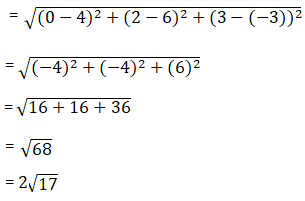Length BC: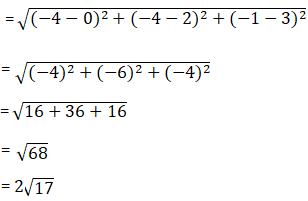Length AC: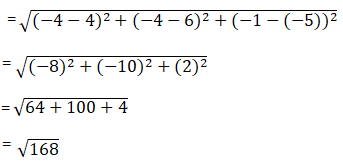From above result, we have AB = BC

Therefore, vertices A, B, C forms an isosceles triangle.

Question 4: Show that the points A(0, 1, 2), B(2, -1, 3) and C(1, -3, 1) are the vertices of an isosceles right-angled triangle.

Solution:

Given points are the vertices of an isosceles right-angled triangle if sum of squares of two sides is equal to square of third side.

Apply distance formula: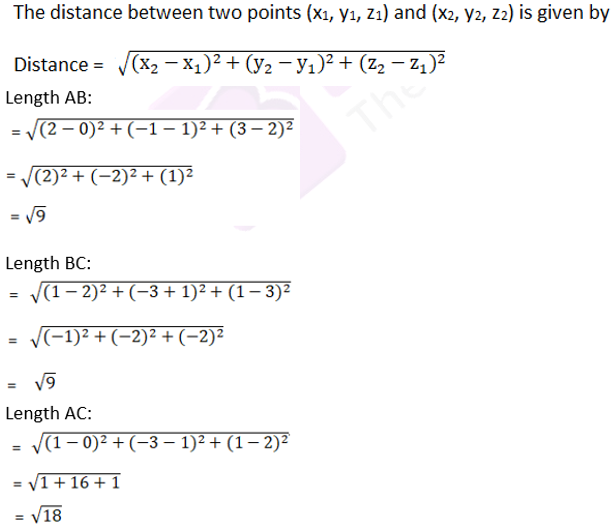Now, AB2 + BC2 = 9 + 9

= 18

= AC2

Therefore, Therefore, vertices A, B, C are the vertices of an isosceles right-angled triangle.

Question 5: Show that the points A(1, 1, 1), B(-2, 4, 1), C(1, -5, 5) and D(2, 2, 5) are the vertices of a square.

Solution:

Points A(1, 1, 1), B(-2, 4, 1), C(1, -5, 5) and D(2, 2, 5) are the vertices of a square if all sides are of equal measure.

Apply distance formula, and find the distance between all the points: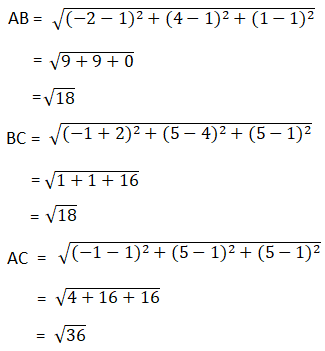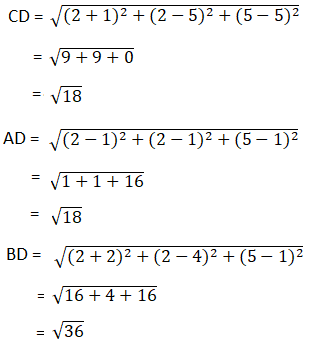From above results, AB = BC = CD = AD, and AC = BD

Hence vertices A, B, C, D form a square.

Question 6: Show that the points A(1, 2, 3), B(-1, -2, -1), C(2, 3, 2) and D(4, 7, 6) are the vertices of a parallelogram. Show that ABCD is not a rectangle.

Solution:

Apply distance formula, and find the distance between all the points: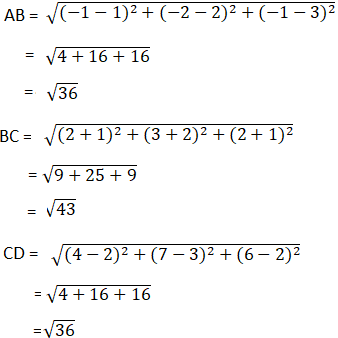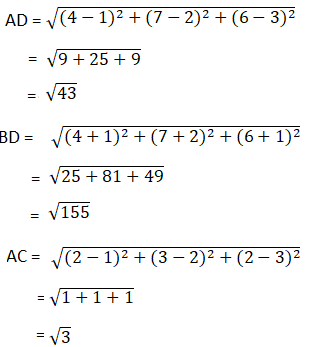From above result, we have

AB = CD [opposite sides]

Diagonal AC ≠ BD, Diagonals are not equal

Hence, Points are vertices of parallelogram and ABCD is not a rectangle.

Question 7: Show that the points P(2, 3, 5), Q(-4, 7, -7), R(-2, 1, -10) and S(4, -3, 2) are the vertices of a rectangle.

Solution:

PQRS is a rectangle if opposite sides are equal and diagonals are also of equal length.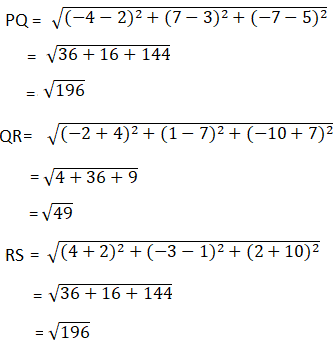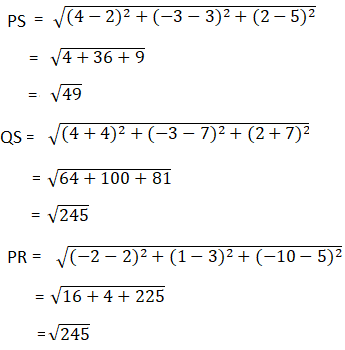Here, PQ = RS and QR = PS and PR = QS

Hence, points are vertices of a rectangle.

Question 8: Show that the points P(1, 3, 4), Q(-1, 6, 10), R(-7, 4, 7) and S(-5, 1, 1) are the vertices of a rhombus.

Solution:

Apply distance formula and find the length of each side.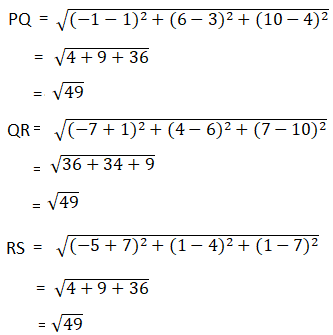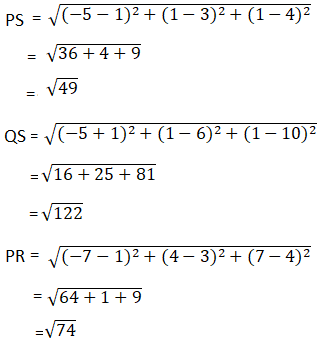=> PQ = RS =QR = PS

And, diagonals: PR ≠ QS

Hence, points are vertices of a rhombus.

## Access other exercise solutions of Class 11 Maths Chapter 26 Three Dimensional Geometry

Exercise 26A Solutions

Exercise 26C Solutions

## R S Aggarwal Solutions for Class 11 Maths Chapter 26 Exercise 26B

Class 11 Maths Chapter 26 Three Dimensional Geometry Exercise 26B is based on topic – Distance Formula.

Students must go through the below points before they attempt this exercise.